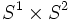# Proving compactness

(diff) ← Older revision | Latest revision (diff) | Newer revision → (diff)
This is a survey article related to:compact space
View other survey articles about compact space

The article is about techniques that one could use to prove that a certain topological space is compact. Compactness is a very useful assumption, and implies a lot in a variety of contexts. However, proving compactness directly from the definitions can be very hard, because the definitions involve starting out with an arbitrary open cover. Typically, proving that a certain space is compact, relies on using the fact that certain other closely related space is compact.

## Key results used

### For general topological spaces

• A product of compact spaces is compact. This result, in the case of arbitrary products, goes by the name of Tychonoff's theorem, though the proof for finite products follows more directly from the tube lemma.
• Any closed subset of a compact space is compact. Further information: compactness is weakly hereditary
• For a fiber bundle with compact base and compact fibers, the total space is compact.
• A finite union of compact subsets of a topological space is compact.

### For metric spaces

For metric spaces, there are other criteria to determine compactness. A metric space is compact iff it is complete and totally bounded i.e. for every$\epsilon > 0$, the space can be expressed as a finite union of$\epsilon$-balls.

### For subsets of Euclidean space

The Heine-Borel theorem tells us that a subset of Euclidean space is compact iff it is closed and bounded. This result essentially hinges on the facts listed for general topological spaces, along with the key fact that the unit interval is compact, as follows:

• The unit interval is compact
• By scaling, any closed bounded interval is compact
• Thus, a product of closed bounded intervals (i.e. a closed bounded rectangle) is compact.
• Any closed and bounded set is contained as a closed subset of closed and bounded intervals. Since any closed subset of a compact space is compact, this completes the proof.

## Proving compactness of manifolds

Often, the method by which a manifold is constructed makes it clear that it is compact. For instance, the manifold$S^1 \times S^2$ is compact, because each of the factors is compact. At other times, the fact that the manifold embeds as a closed and bounded subset of some Euclidean space tells us that it is compact.

Similarly, manifolds constructed as fiber bundles with compact fibers over compact manifolds (for instance, the projectivization of the tangent bundle, or the sphere bundle) are compact.

Another construction that preserves compactness is the connected sum of manifolds. The key reason for this is that the connected sum of two manifolds can be expressed as a union of two subsets, each subset being homeomorphic to a closed subset of one of the original manifold. Thus, combining the fact that closed subsets of compact spaces are compact, and that finite unions of compact subsets are compact, we see that the new manifold we get as a connected sum, is again compact.

Similar reasoning works for other kinds of sums, such as fiber sum over a more complicated submanifold.